Defines a gradient fill. Use a GraphicsGradientFill object with the `Graphics.drawGraphicsData()` method. Drawing a GraphicsGradientFill object is the equivalent of calling the `Graphics.beginGradientFill()` method.

### `new (?type:GradientType, ?colors:Array<Int>, ?alphas:Array<Float>, ?ratios:Array<Int>, ?matrix:Matrix, ?spreadMethod:SpreadMethod, ?interpolationMethod:InterpolationMethod, focalPointRatio:Float = 0)`

Parameters:

`type` A value from the GradientType class that specifies which gradient type to use: `GradientType.LINEAR` or `GradientType.RADIAL`. A transformation matrix as defined by the openfl.geom.Matrix class. The openfl.geom.Matrix class includes a `createGradientBox()` method, which lets you conveniently set up the matrix for use with the `beginGradientFill()` method. A value from the SpreadMethod class that specifies which spread method to use, either: `SpreadMethod.PAD`, `SpreadMethod.REFLECT`, or `SpreadMethod.REPEAT`. A value from the InterpolationMethod class that specifies which value to use: `InterpolationMethod.LINEAR_RGB` or `InterpolationMethod.RGB` A number that controls the location of the focal point of the gradient. A value of 0 sets the focal point in the center. A value of 1 sets the focal point at one border of the gradient circle. A value of -1 sets the focal point at the other border of the gradient circle. A value less than -1 or greater than 1 is rounded to -1 or 1, respectively.

### `alphas:Array<Float>`

An array of alpha values for the corresponding colors in the colors array. Valid values are between 0 and 1. If the value is less than 0, 0 is used. If the value is greater than 1, 1 is used.

### `colors:Array<Int>`

An array of RGB hexadecimal color values to use in the gradient. For example, red is 0xFF0000, blue is 0x0000FF, and so on. You can specify up to 15 colors. For each color, specify a corresponding value in the alphas and ratios properties.

### `focalPointRatio:Float`

A number that controls the location of the focal point of the gradient. A value of 0 sets the focal point in the center. A value of 1 means that the focal point is at one border of the gradient circle.A value of -1 sets the focal point at the other border of the gradient circle. A value of less than -1 or greater than 1 is rounded to -1 or 1, respectively. For example, the following shows a `focalPointRatio` set to 0.75: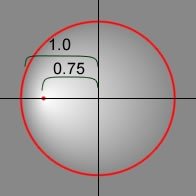### `interpolationMethod:InterpolationMethod`

A value from the InterpolationMethod class that specifies which value to use. Valid values are: `InterpolationMethod.LINEAR_RGB` or `InterpolationMethod.RGB` For example, the following shows a simple linear gradient between two colors (with the `spreadMethod` parameter set to `SpreadMethod.REFLECT`). The different interpolation methods change the appearance as follows:

`InterpolationMethod.LINEAR_RGB``InterpolationMethod.RGB`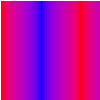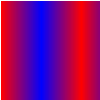### `matrix:Matrix`

A transformation matrix as defined by the Matrix class. The openfl.geom.Matrix class includes a `createGradientBox()` method to set up the matrix for use with the `beginGradientFill()` method.

### `ratios:Array<Int>`

An array of color distribution ratios. Valid values are between 0 and 255. This value defines the percentage of the width where the color is sampled at 100%. The value 0 represents the left position in the gradient box, and the value 255 represents the right position in the gradient box. Note: This value represents positions in the gradient box, not the coordinate space of the final gradient which can be wider or thinner than the gradient box. Specify a value for corresponding to each value in the `colors` property.

For example, for a linear gradient that includes two colors (blue and green) the following example illustrates the placement of the colors in the gradient based on different values in the `ratios` array:

`ratios`Gradient
`[0, 127]`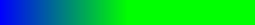`[0, 255]`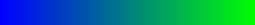`[127, 255]`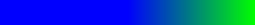The values in the array must increase sequentially; for example, ```[0, 63, 127, 190, 255]```.

### `spreadMethod:SpreadMethod`

A value from the SpreadMethod class that specifies which spread method to use. Valid values are: `SpreadMethod.PAD`, `SpreadMethod.REFLECT`, or `SpreadMethod.REPEAT`.

For example, the following shows a simple linear gradient between two colors:

``````import openfl.geom.*;
import openfl.display.*;

var colors = [0xFF0000, 0x0000FF];
var alphas = [1, 1];
var ratios = [0x00, 0xFF];
var matr = new Matrix();
graphics.drawRect(0,0,100,100);``````

This example uses `SpreadMethod.PAD` for the spread method, and the gradient fill looks like the following: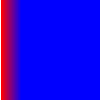If you use `SpreadMethod.REFLECT` for the spread method, the gradient fill looks like the following: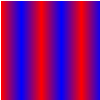If you use `SpreadMethod.REPEAT` for the spread method, the gradient fill looks like the following: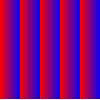### `type:GradientType`

A value from the GradientType class that specifies which gradient type to use. Values are `GradientType.LINEAR` or `GradientType.RADIAL`.